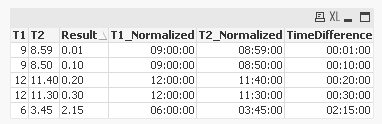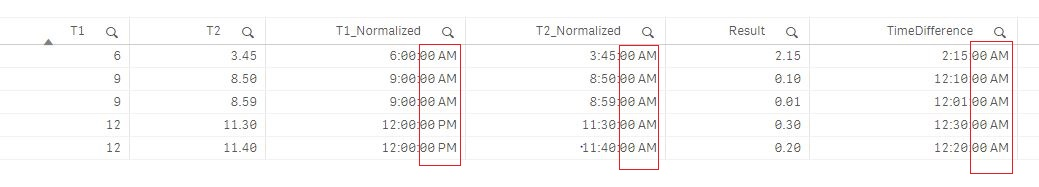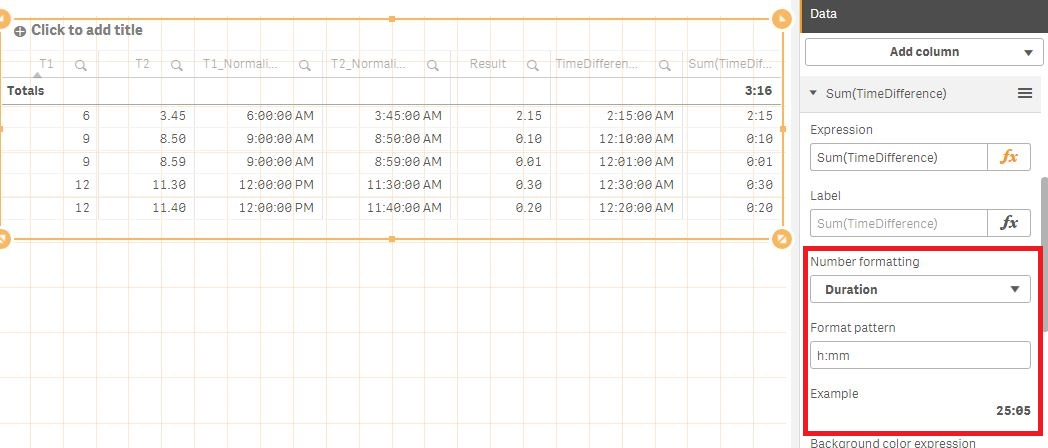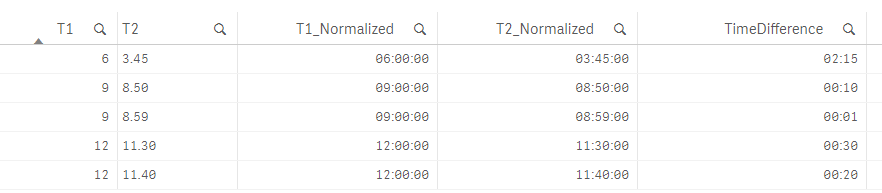# New to Qlik Sense

Discussion board where members can get started with Qlik Sense.

Announcements
Qlik® Product Spotlight: Discover what’s possible. Get more from our products.
See for yourself. Register today.
Contributor II

## How to subtract between two times in qlik sense

Hi Everyone

I need to subtract between two time. Please see the table below

The values in 'T2' shows hours and minutes. For example 8.50 shows 8 hours and 50 minutes. So the difference of T1-T2 should be 9 - 8.50

and the result should be .10 that means the difference is 10 minutes.

I need measure expression to calculate 'Result' field.

T1T2Result
98.500.10
1211.300.30
98.590.01
1211.400.20
63.452.15
1 Solution

Accepted Solutions
Valued Contributor

## Re: How to subtract between two times in qlik sense

Try this code:

```Load *,
Time(T1_Normalized-T2_Normalized) as TimeDifference;
*,
if(substringcount(T2,'.')>0,maketime(mid(T2,1,index(T2,'.')-1),mid(T2,index(T2,'.')+1)),maketime(T2,0)) as T2_Normalized,
if(substringcount(T1,'.')>0,maketime(mid(T1,1,index(T1,'.')-1),mid(T1,index(T1,'.')+1)),maketime(T1,0)) as T1_Normalized
;
load * inline [
T1, T2, Result
9, 8.50, 0.10
12, 11.30, 0.30
9, 8.59, 0.01
12, 11.40, 0.20
6, 3.45, 2.15
];
```5 Replies
Valued Contributor

## Re: How to subtract between two times in qlik sense

Try this code:

```Load *,
Time(T1_Normalized-T2_Normalized) as TimeDifference;
*,
if(substringcount(T2,'.')>0,maketime(mid(T2,1,index(T2,'.')-1),mid(T2,index(T2,'.')+1)),maketime(T2,0)) as T2_Normalized,
if(substringcount(T1,'.')>0,maketime(mid(T1,1,index(T1,'.')-1),mid(T1,index(T1,'.')+1)),maketime(T1,0)) as T1_Normalized
;
load * inline [
T1, T2, Result
9, 8.50, 0.10
12, 11.30, 0.30
9, 8.59, 0.01
12, 11.40, 0.20
6, 3.45, 2.15
];
```Contributor II

## Re: How to subtract between two times in qlik sense

Dear Michele

We are very close enough to the result. I used your script in qlik sense. See the difference in attachment.

I don't need seconds and AM/PM. I only need in HH:MM format.Highlighted
Contributor

## Re: How to subtract between two times in qlik sense

Hi,

Please find attached app, below is the script & table output -

Time:

T2,

Time(T1-T2,'hh-mm') as Diff

FROM

(ooxml, embedded labels, table is Sheet1);

Regards,

Rahul

Contributor II

## Re: How to subtract between two times in qlik sense

Hi Michele

I got the solution.

I changed the number formatting as duration.

Its working fine

Thanks for assisting meValued Contributor

## Re: How to subtract between two times in qlik sense

```Load *,
interval(T1_Normalized-T2_Normalized,'hh:mm') as TimeDifference;
*,
if(substringcount(T2,'.')>0,maketime(mid(T2,1,index(T2,'.')-1),mid(T2,index(T2,'.')+1)),maketime(T2,0)) as T2_Normalized,
if(substringcount(T1,'.')>0,maketime(mid(T1,1,index(T1,'.')-1),mid(T1,index(T1,'.')+1)),maketime(T1,0)) as T1_Normalized
;
load * inline [
T1, T2, Result
9, 8.50, 0.10
12, 11.30, 0.30
9, 8.59, 0.01
12, 11.40, 0.20
6, 3.45, 2.15
];
```Community Browser